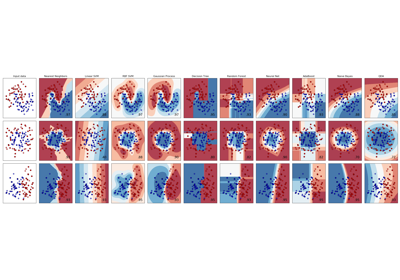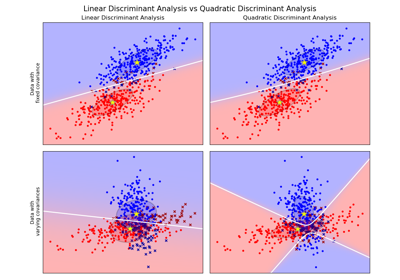# sklearn.discriminant_analysis.QuadraticDiscriminantAnalysis¶

class sklearn.discriminant_analysis.QuadraticDiscriminantAnalysis(priors=None, reg_param=0.0, store_covariance=False, tol=0.0001)[source]

A classifier with a quadratic decision boundary, generated by fitting class conditional densities to the data and using Bayes’ rule.

The model fits a Gaussian density to each class.

Read more in the User Guide.

Parameters
priorsarray, optional, shape = [n_classes]

Priors on classes

reg_paramfloat, optional

Regularizes the covariance estimate as (1-reg_param)*Sigma + reg_param*np.eye(n_features)

store_covarianceboolean

If True the covariance matrices are computed and stored in the self.covariance_ attribute.

New in version 0.17.

tolfloat, optional, default 1.0e-4

Threshold used for rank estimation.

New in version 0.17.

Attributes
covariance_list of array-like of shape (n_features, n_features)

Covariance matrices of each class.

means_array-like of shape (n_classes, n_features)

Class means.

priors_array-like of shape (n_classes)

Class priors (sum to 1).

rotations_list of arrays

For each class k an array of shape [n_features, n_k], with n_k = min(n_features, number of elements in class k) It is the rotation of the Gaussian distribution, i.e. its principal axis.

scalings_list of arrays

For each class k an array of shape [n_k]. It contains the scaling of the Gaussian distributions along its principal axes, i.e. the variance in the rotated coordinate system.

classes_array-like, shape (n_classes,)

Unique class labels.

Examples

>>> from sklearn.discriminant_analysis import QuadraticDiscriminantAnalysis
>>> import numpy as np
>>> X = np.array([[-1, -1], [-2, -1], [-3, -2], [1, 1], [2, 1], [3, 2]])
>>> y = np.array([1, 1, 1, 2, 2, 2])
>>> clf.fit(X, y)
>>> print(clf.predict([[-0.8, -1]]))



Methods

 decision_function(self, X) Apply decision function to an array of samples. fit(self, X, y) Fit the model according to the given training data and parameters. get_params(self[, deep]) Get parameters for this estimator. predict(self, X) Perform classification on an array of test vectors X. predict_log_proba(self, X) Return posterior probabilities of classification. predict_proba(self, X) Return posterior probabilities of classification. score(self, X, y[, sample_weight]) Return the mean accuracy on the given test data and labels. set_params(self, \*\*params) Set the parameters of this estimator.
__init__(self, priors=None, reg_param=0.0, store_covariance=False, tol=0.0001)[source]

Initialize self. See help(type(self)) for accurate signature.

decision_function(self, X)[source]

Apply decision function to an array of samples.

Parameters
Xarray-like of shape (n_samples, n_features)

Array of samples (test vectors).

Returns
Cndarray of shape (n_samples,) or (n_samples, n_classes)

Decision function values related to each class, per sample. In the two-class case, the shape is [n_samples,], giving the log likelihood ratio of the positive class.

fit(self, X, y)[source]

Fit the model according to the given training data and parameters.

Changed in version 0.19: store_covariances has been moved to main constructor as store_covariance

Changed in version 0.19: tol has been moved to main constructor.

Parameters
Xarray-like of shape (n_samples, n_features)

Training vector, where n_samples is the number of samples and n_features is the number of features.

yarray, shape = [n_samples]

Target values (integers)

get_params(self, deep=True)[source]

Get parameters for this estimator.

Parameters
deepbool, default=True

If True, will return the parameters for this estimator and contained subobjects that are estimators.

Returns
paramsmapping of string to any

Parameter names mapped to their values.

predict(self, X)[source]

Perform classification on an array of test vectors X.

The predicted class C for each sample in X is returned.

Parameters
Xarray-like of shape (n_samples, n_features)
Returns
Cndarray of shape (n_samples,)
predict_log_proba(self, X)[source]

Return posterior probabilities of classification.

Parameters
Xarray-like of shape (n_samples, n_features)

Array of samples/test vectors.

Returns
Cndarray of shape (n_samples, n_classes)

Posterior log-probabilities of classification per class.

predict_proba(self, X)[source]

Return posterior probabilities of classification.

Parameters
Xarray-like of shape (n_samples, n_features)

Array of samples/test vectors.

Returns
Cndarray of shape (n_samples, n_classes)

Posterior probabilities of classification per class.

score(self, X, y, sample_weight=None)[source]

Return the mean accuracy on the given test data and labels.

In multi-label classification, this is the subset accuracy which is a harsh metric since you require for each sample that each label set be correctly predicted.

Parameters
Xarray-like of shape (n_samples, n_features)

Test samples.

yarray-like of shape (n_samples,) or (n_samples, n_outputs)

True labels for X.

sample_weightarray-like of shape (n_samples,), default=None

Sample weights.

Returns
scorefloat

Mean accuracy of self.predict(X) wrt. y.

set_params(self, **params)[source]

Set the parameters of this estimator.

The method works on simple estimators as well as on nested objects (such as pipelines). The latter have parameters of the form <component>__<parameter> so that it’s possible to update each component of a nested object.

Parameters
**paramsdict

Estimator parameters.

Returns
selfobject

Estimator instance.

## Examples using sklearn.discriminant_analysis.QuadraticDiscriminantAnalysis¶